# 4.1 Linear functions  (Page 9/27)

 Page 9 / 27

## Vertical stretch or compression

In the equation $\text{\hspace{0.17em}}f\left(x\right)=mx,$ the $\text{\hspace{0.17em}}m\text{\hspace{0.17em}}$ is acting as the vertical stretch    or compression of the identity function. When $\text{\hspace{0.17em}}m\text{\hspace{0.17em}}$ is negative, there is also a vertical reflection of the graph. Notice in [link] that multiplying the equation of $\text{\hspace{0.17em}}f\left(x\right)=x\text{\hspace{0.17em}}$ by $\text{\hspace{0.17em}}m\text{\hspace{0.17em}}$ stretches the graph of $\text{\hspace{0.17em}}f\text{\hspace{0.17em}}$ by a factor of $\text{\hspace{0.17em}}m\text{\hspace{0.17em}}$ units if $\text{\hspace{0.17em}}m>\text{1}\text{\hspace{0.17em}}$ and compresses the graph of $\text{\hspace{0.17em}}f\text{\hspace{0.17em}}$ by a factor of $\text{\hspace{0.17em}}m\text{\hspace{0.17em}}$ units if $\text{\hspace{0.17em}}0 This means the larger the absolute value of $\text{\hspace{0.17em}}m,\text{\hspace{0.17em}}$ the steeper the slope.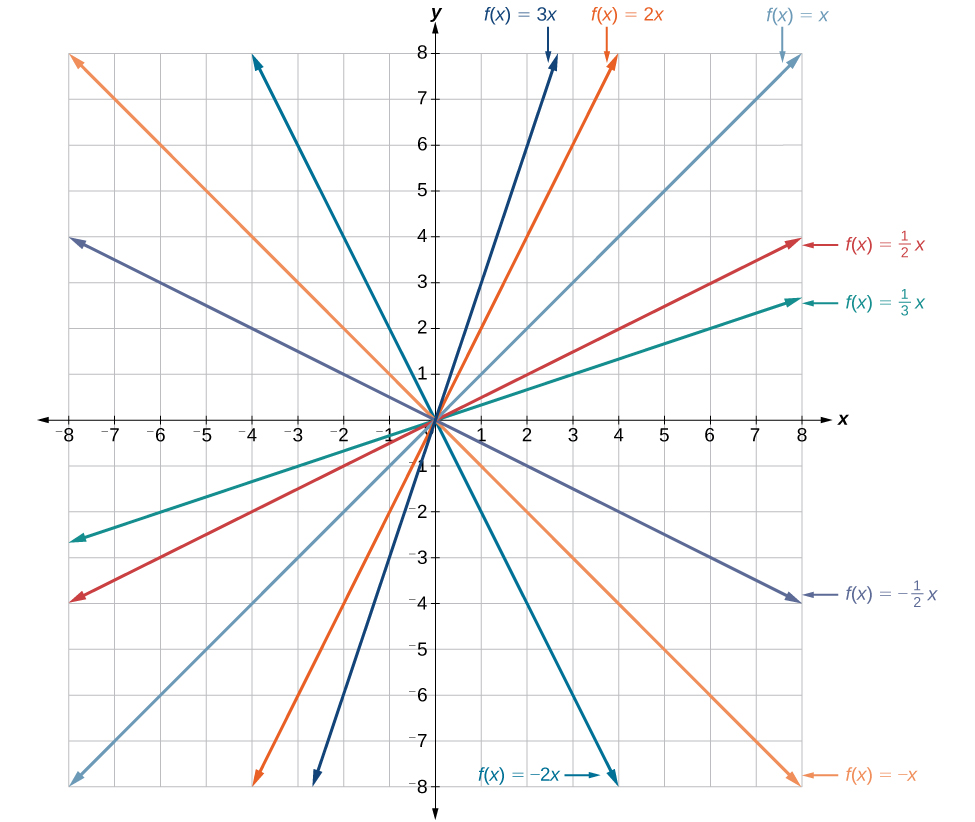Vertical stretches and compressions and reflections on the function   f ( x ) = x

## Vertical shift

In $\text{\hspace{0.17em}}f\left(x\right)=mx+b,$ the $\text{\hspace{0.17em}}b\text{\hspace{0.17em}}$ acts as the vertical shift    , moving the graph up and down without affecting the slope of the line. Notice in [link] that adding a value of $\text{\hspace{0.17em}}b\text{\hspace{0.17em}}$ to the equation of $\text{\hspace{0.17em}}f\left(x\right)=x\text{\hspace{0.17em}}$ shifts the graph of $\text{\hspace{0.17em}}f\text{\hspace{0.17em}}$ a total of $\text{\hspace{0.17em}}b\text{\hspace{0.17em}}$ units up if $\text{\hspace{0.17em}}b\text{\hspace{0.17em}}$ is positive and $\text{\hspace{0.17em}}|b|\text{\hspace{0.17em}}$ units down if $\text{\hspace{0.17em}}b\text{\hspace{0.17em}}$ is negative.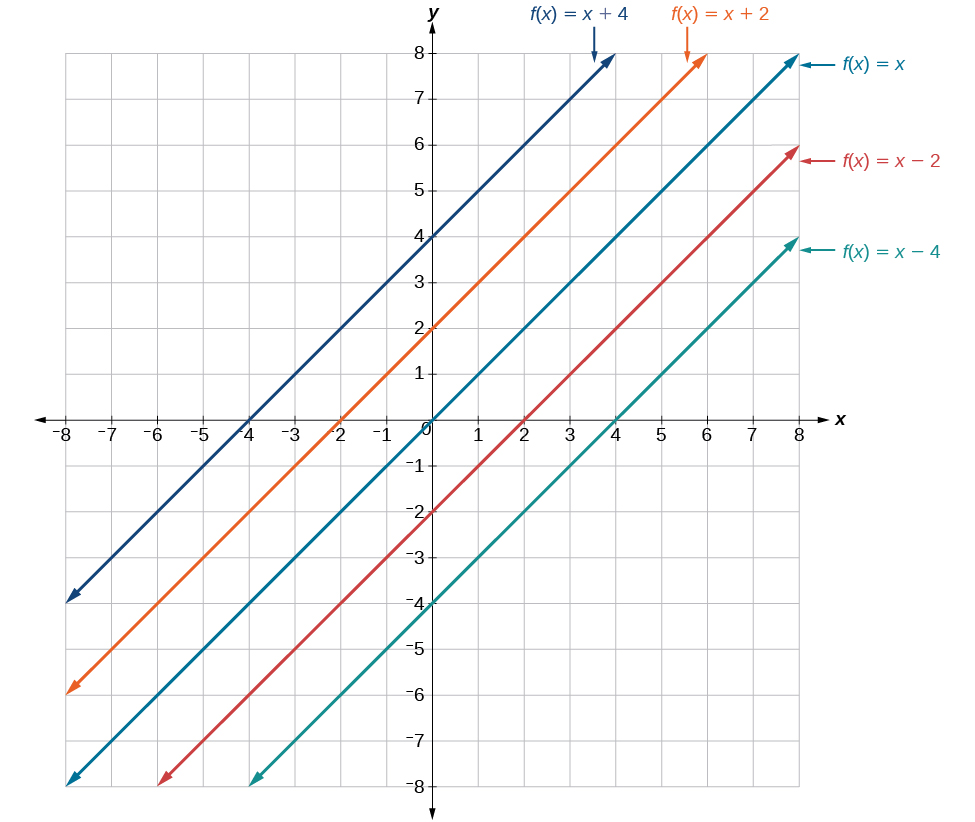This graph illustrates vertical shifts of the function f ( x ) = x .

Using vertical stretches or compressions along with vertical shifts is another way to look at identifying different types of linear functions. Although this may not be the easiest way to graph this type of function, it is still important to practice each method.

Given the equation of a linear function, use transformations to graph the linear function in the form $\text{\hspace{0.17em}}f\left(x\right)=mx+b.$

1. Graph $\text{\hspace{0.17em}}f\left(x\right)=x.$
2. Vertically stretch or compress the graph by a factor $\text{\hspace{0.17em}}m.$
3. Shift the graph up or down $\text{\hspace{0.17em}}b\text{\hspace{0.17em}}$ units.

## Graphing by using transformations

Graph $\text{\hspace{0.17em}}f\left(x\right)=\frac{1}{2}x-3\text{\hspace{0.17em}}$ using transformations.

The equation for the function shows that $\text{\hspace{0.17em}}m=\frac{1}{2}\text{\hspace{0.17em}}$ so the identity function is vertically compressed by $\text{\hspace{0.17em}}\frac{1}{2}.\text{\hspace{0.17em}}$ The equation for the function also shows that $\text{\hspace{0.17em}}b=-3\text{\hspace{0.17em}}$ so the identity function is vertically shifted down 3 units. First, graph the identity function, and show the vertical compression as in [link] .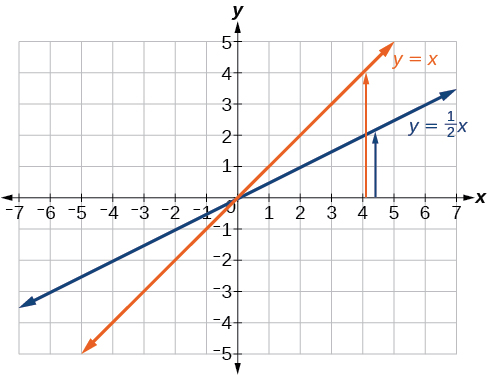The function,   y = x , compressed by a factor of   1 2

Then show the vertical shift as in [link] .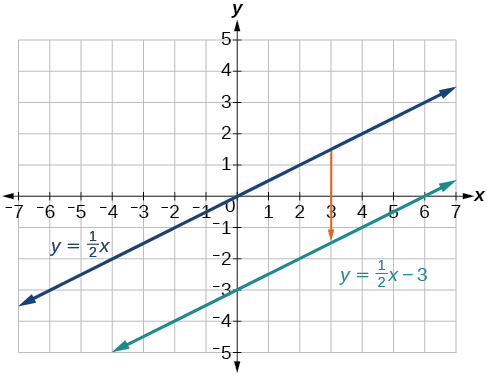The function   y = 1 2 x , shifted down 3 units

Graph $\text{\hspace{0.17em}}f\left(x\right)=4+2x\text{\hspace{0.17em}}$ using transformations.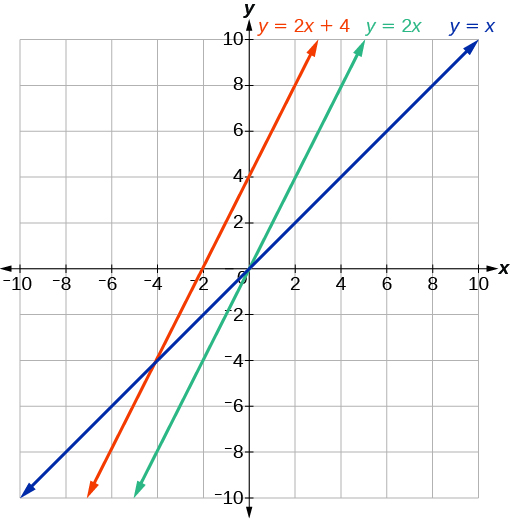In [link] , could we have sketched the graph by reversing the order of the transformations?

No. The order of the transformations follows the order of operations. When the function is evaluated at a given input, the corresponding output is calculated by following the order of operations. This is why we performed the compression first. For example, following the order: Let the input be 2.

$\begin{array}{ccc}\hfill f\left(2\right)& =& \frac{1}{2}\left(2\right)-3\hfill \\ & =& 1-3\hfill \\ & =& -2\hfill \end{array}$

## Writing the equation for a function from the graph of a line

Earlier, we wrote the equation for a linear function from a graph. Now we can extend what we know about graphing linear functions to analyze graphs a little more closely. Begin by taking a look at [link] . We can see right away that the graph crosses the y -axis at the point $\text{\hspace{0.17em}}\left(0,\text{4}\right)\text{\hspace{0.17em}}$ so this is the y -intercept.

Then we can calculate the slope by finding the rise and run. We can choose any two points, but let’s look at the point $\text{\hspace{0.17em}}\left(–2,0\right).\text{\hspace{0.17em}}$ To get from this point to the y- intercept, we must move up 4 units (rise) and to the right 2 units (run). So the slope must be

$m=\frac{\text{rise}}{\text{run}}=\frac{4}{2}=2$

Substituting the slope and y- intercept into the slope-intercept form of a line gives

how do I set up the problem?
what is a solution set?
Harshika
find the subring of gaussian integers?
Rofiqul
hello, I am happy to help!
Abdullahi
hi mam
Mark
find the value of 2x=32
divide by 2 on each side of the equal sign to solve for x
corri
X=16
Michael
Want to review on complex number 1.What are complex number 2.How to solve complex number problems.
Beyan
yes i wantt to review
Mark
use the y -intercept and slope to sketch the graph of the equation y=6x
how do we prove the quadratic formular
Darius
hello, if you have a question about Algebra 2. I may be able to help. I am an Algebra 2 Teacher
thank you help me with how to prove the quadratic equation
Seidu
may God blessed u for that. Please I want u to help me in sets.
Opoku
what is math number
4
Trista
x-2y+3z=-3 2x-y+z=7 -x+3y-z=6
can you teacch how to solve that🙏
Mark
Solve for the first variable in one of the equations, then substitute the result into the other equation. Point For: (6111,4111,−411)(6111,4111,-411) Equation Form: x=6111,y=4111,z=−411x=6111,y=4111,z=-411
Brenna
(61/11,41/11,−4/11)
Brenna
x=61/11 y=41/11 z=−4/11 x=61/11 y=41/11 z=-4/11
Brenna
Need help solving this problem (2/7)^-2
x+2y-z=7
Sidiki
what is the coefficient of -4×
-1
Shedrak
the operation * is x * y =x + y/ 1+(x × y) show if the operation is commutative if x × y is not equal to -1
An investment account was opened with an initial deposit of \$9,600 and earns 7.4% interest, compounded continuously. How much will the account be worth after 15 years?
lim x to infinity e^1-e^-1/log(1+x)
given eccentricity and a point find the equiationByByBy OpenStaxBy John GabrieliByBy Sheila LopezBy Danielle StephensBy Madison ChristianBy Ali SidBy John GabrieliBy Mary MateraBy OpenStax